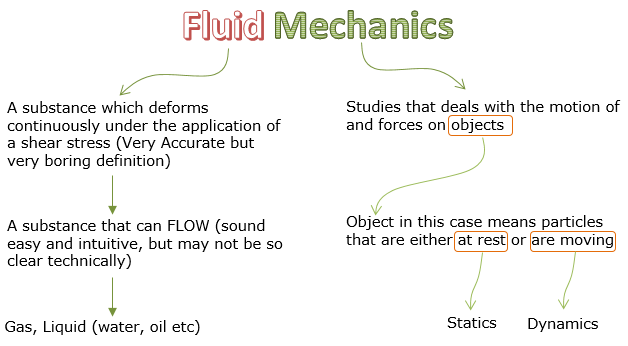Mechanical Engineering - Fluid Mechanics                                 Home : www.sharetechnote.com Fluid Mechanics : Introduction   Fluid Mechanics is the study of fluid flow that is used to analysis of various application that operate based on liquid body such as power turbines/pumps. Liquids are used to measure pressure of a moving fluid. In fluid mechanics, you also do rigid-body equilibrium analysis where fluids exert force on the system. Like many other studies, you can get pretty detailed / clear understandings on the meaning of 'Fluid Mechanics' just by analyzing each word as below.Fluid Mechanics is one of the most challenging courses in engineering that Involves heavy-scale of calculations, starting from combining and rearranging equations – number of problems require more than one equation to solve for a variable     Common Applications   Some of the applications to which Fluid Mechanics are applied are as follows : Analyzing the flow of water going through the piping system for household usage Calculating the reaction forces and moments onto an object where fluid(s) flow into Calculating the magnitude of forces acting on an object submerged into a liquid Analyzing the performance of pump/turbine in terms of power